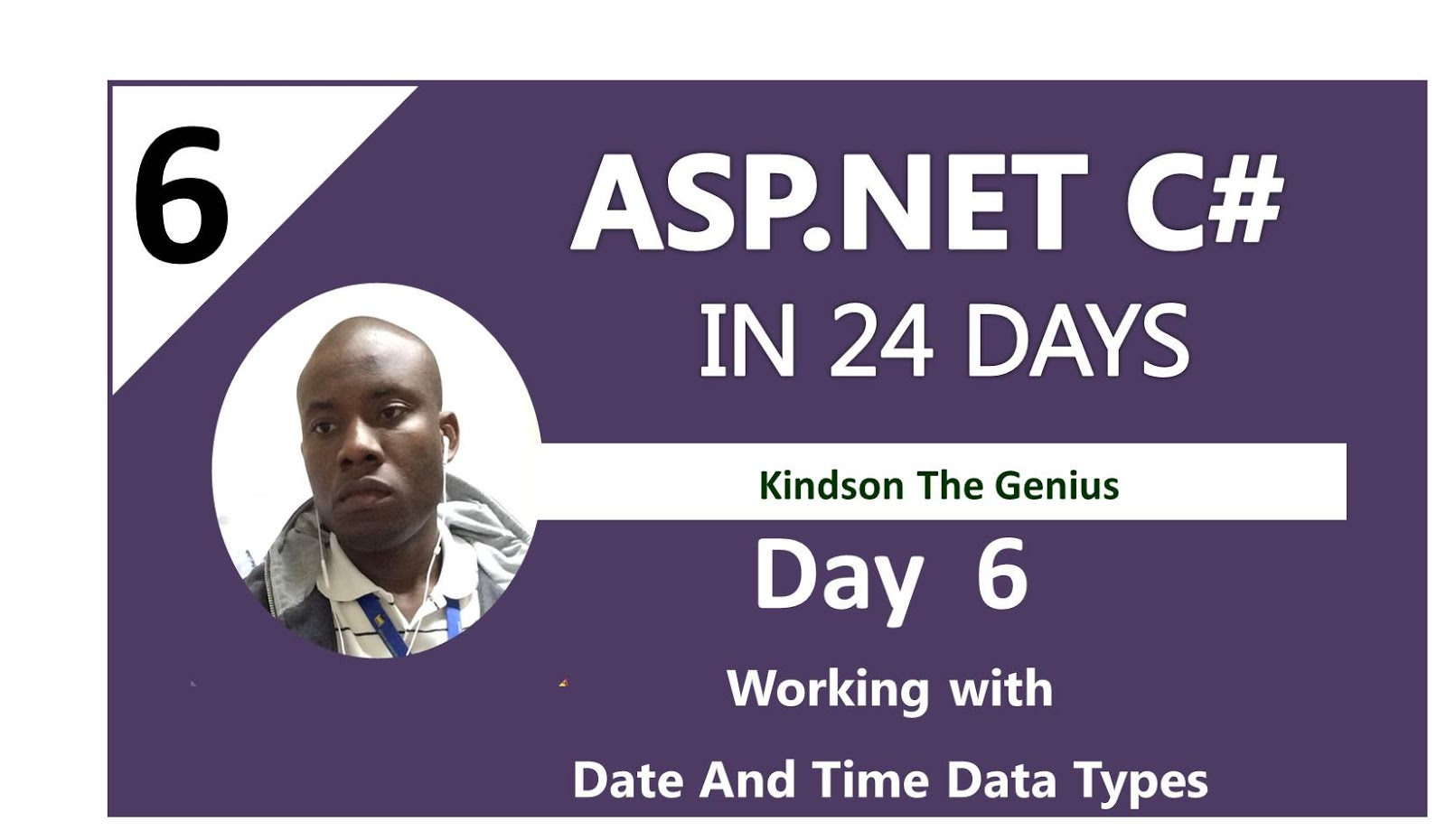# Day 6: Handling Date and Time Data Types in C#Hello, good to know that you are making fast progress.
Welcome to day 6 of ASP.NET in 24 days!

Today, we are going the learn how to handle date and time data types in C# as well as conversions.

We would cover the following

Note: To run the programs in this lesson, you need to copy the code and use it to replace the main function.

### 1. Using DateTime type

In C# a DateTime data type is a struct type that represents an instant of time.
The DateTime is a value type that represents dates and times values that range from:
00:00:00, January 1, 0001 to
11:59:59, December 31, 9999

A particular data is the number of ticks since 00:00:00 January 1, 0001. This are just some basic theory you need to just have in mind

### 2. Creating an new date using the DateTime constructor

• You can create a new datetime object in any of the following ways:
• Calling the DateTime constructor and specifying the arguments
• Converting a string representation to a datetime using inbuilt functions

You can test these two methods using the code below.Create a console application, then copy and run the code snippet below.

### 3. Declaring date data type

To declare a DateTime object, you simply using the sytax

DateTime mydate = new DateTime()

In this case, the variable mydate is declared as a DateTime object but has not been assigned a value yet.

### 4. Converting to a date using Convert.toDateTime()

One of the ways to convert a value to a DateTime value is using the Convert.ToDateTime() function. I used this method most of the time.
You can basically convert any other type to DateTime() type. Run the following code snippet to see how it works.

```static void Main(string[] args)
{
Program pr = new Program();
pr.UsingConvert();
}
public void UsingConvert()
{
DateTime mydate = Convert.ToDateTime(1000);
Console.WriteLine();

}
```

Listing 1.0: Using the Convert.ToDate() function (doesn’t work!)

It doesn’t work! So don’t use it
It is however included in the C# documentation;

### 5. Converting Date to a String

You can convert a string expression to a DateTime type using any of the following:
Parse(): Converts a string to its equivalent DateTime value
ParseExact(): This function converts a string to DateTime, but the string needs to match the exact date format, or else, there would be an error.
TryParse(): Converts string to DateTime but returns a value to indicate if the conversion succeeded or not.
TryParseExact: Converts a string to DateTime. The string format much match the date format and also value is returned to indicate success or failure

Run The code snippet below and try to understand how it works

```static void Main(string[] args)
{
Program pr = new Program();
pr.UsingParse();
}
public void UsingParse()
{
string dateInput = "Jan 1, 2018";
DateTime myDate = DateTime.Parse(dateInput);
Console.WriteLine(myDate);
// DISPLAYS THE DATE IN THE FORMATE 1/1/2018 12:00:00 AM
}
```

Listing 2.0: Using the Parse Function

The listing 2.0 shows the conversion to DateTime() using Parse().
Quiz: Try to modify the code to use other Parse functions you have learnt here.

### 6. Getting Today’s date and time

There are many ways you can get the current date and time using the C# code:

• DateTime.Today: Returns the current date
• DateTime.Utc.Now.Date: Returns the current date
• DateTime.Now.ToString(“HH:mm:ss”);
• DateTime.Today.ToString(“dd-MM-yyyy”);

Try out all this methods by running the code snippet below.

```static void Main(string[] args)
{
Program pr = new Program();
pr.WorkingWithDate();
}
public void WorkingWithDate()
{
Console.WriteLine(DateTime.Today); //Returns the current date
Console.WriteLine(DateTime.UtcNow.Date); //Returns the current date
Console.WriteLine(DateTime.Now.ToString("HH:mm:ss"));
Console.WriteLine(DateTime.Today.ToString("dd-MM-yyyy"));
DateTime.Now.ToString("yyyy-MM-dd hh:mm:ss");
}
```

Listing 3.0: Getting Current Date and Time

### 7. Extracting Day, Month and Year from Date

You can extract the day, month or year part of a given date. When they are extracted, you can use then either as string or any other datatype. Here we assume we extract them as string.
So, the Listing 4.0, gives the code snippet that does the splitting. It is very clear and easy to understand. Run it and make sure you understands how it works.

```static void Main(string[] args)
{
Program pr = new Program();
pr.SplitingDates();
}
public void SplitingDates()
{
String myDate = DateTime.Now.ToString(); //GET THE CURRENT DATE
DateTime datevalue = (Convert.ToDateTime(myDate.ToString())); //CONVERTS THE myDate to a      DateTime TYPE

String dy = datevalue.Day.ToString();
String mn = datevalue.Month.ToString();
String yr = datevalue.Year.ToString();

Console.WriteLine("The day is: " + dy);
Console.WriteLine("The month is: " + mn);
Console.WriteLine("The year is: " + yr);
}
```

Listing 4.0: Extracting Day, Month and Year from a date

### 8. About TimeSpan Data Type

According the the msdn documentation, a TimeSpan represents a time interval.
Think of TimeSpan as a diffrence in time. When you subtract or add two dates, the result you have will be a TimeSpan. That is why it is called ‘a time interval’.

Just like a Date contains day, month and year, a TimeSpan contains the following:

• Days
• Hours
• Milliseconds
• Minutes
• Second

The Hours property of TimeSpan represents whole hours, while the TotalHours property represents whole and fractional hours of the TimeSpan.
How TimeSpan works is illustrated in Listing 5.0. Please try to make sure you copy and run the code:

```static void Main(string[] args)
{
Program pr = new Program();
pr.UsingTimeSpan();
}
public void UsingTimeSpan()
{
TimeSpan span = DateTime.Now - DateTime.Now.Date;
int days = span.Days;
int hours = span.Hours;
int mins = span.Minutes;
int seconds = span.Seconds;
int milisecs = span.Milliseconds;

Console.WriteLine("Days: " + days);
Console.WriteLine("Hours: " + hours);
Console.WriteLine("Minutes: " + mins);
Console.WriteLine("Seconds: " + seconds);
Console.WriteLine("Milliseconds: " + milisecs);
}
```

Listing 5.0: Using TimeSpan in C#

### 9. Final Note

Congrats if you have come this far!
If you encountered any challenges implementing it in the comment box below and someone would give you the needed assistance.